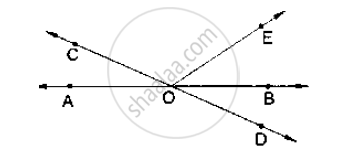# In Below Figure, Lines Ab and Cd Intersect at O. If ∠Aoc + ∠Boe = 70° and ∠Bod = 40°, Find ∠Boe and Reflex ∠Coe. - Mathematics

In below figure, lines AB and CD intersect at O. If ∠AOC + ∠BOE = 70° and ∠BOD =
40°, find ∠BOE and reflex ∠COE.#### Solution

Given that

∠AOC + ∠BOE = 70° and ∠BOD = 40°

∠BOE = ?

Here,  ∠BOD and  ∠AOC are vertically opposite angles

∠BOD = ∠AOC = 40°

Given ∠AOC + ∠BOE = 70°

40° + ∠BOF = 70°

∠BOF = 70° - 40°

∠BOE = 30°

∠AOC and ∠BOC are linear pair of angles

⇒∠AOC + ∠COF + ∠BOE = 180°

⇒ ∠COE = 180° - 30° - 40°

⇒ ∠COE = 110°

∴Reflex ∠COE = 360° -110° = 250°.

Concept: Pairs of Angles
Is there an error in this question or solution?

#### APPEARS IN

RD Sharma Mathematics for Class 9
Chapter 10 Lines and Angles
Exercise 10.3 | Q 9 | Page 23